## Introduction

`qgcomp` is a package to implement g-computation for analyzing the effects of exposure mixtures. Quantile g-computation yields estimates of the effect of increasing all exposures by one quantile, simultaneously. This, it estimates a “mixture effect” useful in the study of exposure mixtures such as air pollution, diet, and water contamination.

Using terminology from methods developed for causal effect estimation, quantile g-computation estimates the parameters of a marginal structural model that characterizes the change in the expected potential outcome given a joint intervention on all exposures, possibly conditional on confounders. Under the assumptions of exchangeability, causal consistency, positivity, no interference, and correct model specification, this model yields a causal effect for an intervention on the mixture as a whole. While these assumptions may not be met exactly, they provide a useful roadmap for how to interpret the results of a qgcomp fit, and where efforts should be spent in terms of ensuring accurate model specification and selection of exposures that are sufficient to control co-pollutant confounding.

## How to use the `qgcomp` package

Here we use a running example from the `metals` dataset from the from the package `qgcomp` to demonstrate some features of the package and method.

Namely, the examples below demonstrate use of the package for: 1. Fast estimation of exposure effects under a linear model for quantized exposures for continuous (normal) outcomes 2. Estimating conditional and marginal odds/risk ratios of a mixture effect for binary outcomes 3. Adjusting for non-exposure covariates when estimating effects of the mixture 4. Allowing non-linear and non-homogenous effects of individual exposures and the mixture as a whole by including product terms 5. Using qgcomp to fit a time-to-event model to estimate conditional and marginal hazard ratios for the exposure mixture

For analogous approaches to estimating exposure mixture effects, illustrative examples can be seen in the `gQWS` package help files, which implements weighte quantile sum (WQS) regression, and at https://jenfb.github.io/bkmr/overview.html, which describes Bayesian kernel machine regression.

The `metals` dataset from the from the package `qgcomp`, comprises a set of simulated well water exposures and two health outcomes (one continuous, one binary/time-to-event). The exposures are transformed to have mean = 0.0, standard deviation = 1.0. The data are used throughout to demonstrate usage and features of the `qgcomp` package.

``````library("qgcomp")
library("knitr")
library("ggplot2")
data("metals", package="qgcomp")
head(metals)
``````
``````##       arsenic      barium    cadmium    calcium    chloride    chromium
## 1  0.09100165  0.08166362 15.0738845 -0.7746662 -0.15408335 -0.05589104
## 2  0.17018302 -0.03598828 -0.7126486 -0.6857886 -0.19605499 -0.03268488
## 3  0.13336869  0.09934014  0.6441992 -0.1525231 -0.17511844 -0.01161098
## 4 -0.52570747 -0.76616263 -0.8610256  1.4472733  0.02552401 -0.05173287
## 5  0.43420529  0.40629920  0.0570890  0.4103682 -0.24187403 -0.08931824
## 6  0.71832662  0.19559582 -0.6823437 -0.8931696 -0.03919936 -0.07389407
##        copper       iron         lead  magnesium   manganese     mercury
## 1  1.99438050 19.1153352 21.072630908 -0.5109546  2.07630966 -1.20826726
## 2 -0.02490169 -0.2039425 -0.010378362 -0.1030542 -0.36095395 -0.68729723
## 3  0.25700811 -0.1964581 -0.063375935  0.9166969 -0.31075240  0.44852503
## 4  0.75477075 -0.2317787 -0.002847991  2.5482987 -0.23350205  0.20428158
## 5 -0.09919923 -0.1698619 -0.035276281 -0.5109546  0.08825996  1.19283834
## 6 -0.05622285 -0.2129300 -0.118460981 -1.0059145 -0.30219838  0.02875033
##      nitrate    nitrite         ph    selenium     silver      sodium
## 1  1.3649492 -1.0500539 -0.7125482  0.23467592 -0.8648653 -0.41840695
## 2 -0.1478382  0.4645119  0.9443009  0.65827253 -0.8019173 -0.09112969
## 3 -0.3001660 -1.4969868  0.4924330  0.07205576 -0.3600140 -0.11828963
## 4  0.3431814 -0.6992263 -0.4113029  0.23810705  1.3595205 -0.11828963
## 5  0.0431269 -0.5041390  0.3418103 -0.02359910 -1.6078044 -0.40075299
## 6 -0.3986575  0.1166249  1.2455462 -0.61186017  1.3769466  1.83722597
##      sulfate total_alkalinity total_hardness       zinc mage35          y
## 1 -0.1757544      -1.31353389    -0.85822417  1.0186058      1 -0.6007989
## 2 -0.1161359      -0.12699789    -0.67749970 -0.1509129      0 -0.2022296
## 3 -0.1616806       0.42671890     0.07928399 -0.1542524      0 -1.2164116
## 4  0.8272415       0.99173604     1.99948142  0.1843372      0  0.1826311
## 5 -0.1726845      -0.04789549     0.30518957 -0.1529379      0  1.1760472
## 6 -0.1385631       1.98616621    -1.07283447 -0.1290391      0 -0.4100912
##   disease_time disease_state
## 1 6.168764e-07             1
## 2 4.000000e+00             0
## 3 4.000000e+00             0
## 4 4.000000e+00             0
## 5 1.813458e+00             1
## 6 2.373849e+00             1
``````

### Example 1: linear model

``````# we save the names of the mixture variables in the variable "Xnm"
Xnm <- c(
'arsenic','barium','cadmium','calcium','chromium','copper',
'iron','lead','magnesium','manganese','mercury','selenium','silver',
'sodium','zinc'
)
covars = c('nitrate','nitrite','sulfate','ph', 'total_alkalinity','total_hardness')

# Example 1: linear model
# Run the model and save the results "qc.fit"
system.time(qc.fit <- qgcomp.noboot(y~.,dat=metals[,c(Xnm, 'y')], family=gaussian()))
``````
``````## Including all model terms as exposures of interest
``````
``````##    user  system elapsed
##   0.022   0.002   0.024
``````
``````#   user  system elapsed
#  0.011   0.002   0.018

# contrasting other methods with computational speed
# WQS regression
#system.time(wqs.fit <- gwqs(y~NULL,mix_name=Xnm, data=metals[,c(Xnm, 'y')], family="gaussian"))
#   user  system elapsed
# 35.775   0.124  36.114

# Bayesian kernel machine regression (note that the number of iterations here would
#  need to be >5,000, at minimum, so this underestimates the run time by a factor
#  of 50+
#system.time(bkmr.fit <- kmbayes(y=metals\$y, Z=metals[,Xnm], family="gaussian", iter=100))
#   user  system elapsed
# 81.644   4.194  86.520

#first note that qgcomp is very fast

# View results: scaled coefficients/weights and statistical inference about
# mixture effect
qc.fit
``````
``````## Scaled effect size (positive direction, sum of positive coefficients = 0.39)
##  calcium     iron   barium   silver  arsenic  mercury   sodium chromium
##  0.72216  0.06187  0.05947  0.03508  0.03447  0.02451  0.02162  0.02057
##  cadmium     zinc
##  0.01328  0.00696
##
## Scaled effect size (negative direction, sum of negative coefficients = -0.124)
## magnesium    copper      lead manganese  selenium
##  0.475999  0.385299  0.074019  0.063828  0.000857
##
## Mixture slope parameters (Delta method CI):
##
##              Estimate Std. Error Lower CI Upper CI t value
## (Intercept) -0.356670   0.107878 -0.56811 -0.14523   1e-03
## psi1         0.266394   0.071025  0.12719  0.40560   2e-04
``````

One advantage of quantile g-computation over other methods that estimate “mixture effects” (the effect of changing all exposures at once), is that it is very computationally efficient. Contrasting methods such as WQS (`gWQS` package) and Bayesian Kernel Machine regression (`bkmr` package), quantile g-computation can provide results many orders of magnitude faster. For example, the example above ran 3000X faster for quantile g-computation versus WQS regression, and we estimate the speedup would be several hundred thousand times versus Bayesian kernel machine regression.

Quantile g-computation yields fixed weights in the estimation procedure, similar to WQS regression. However, note that the weights from `qgcomp.noboot` can be negative or positive. When all effects are linear and in the same direction (“directional homogeneity”), quantile g-computation is equivalent to weighted quantile sum regression in large samples.

The overall mixture effect from quantile g-computation (psi1) is interpreted as the effect on the outcome of increasing every exposure by one quantile, possibly conditional on covariates. Given the overall exposure effect, the weights are considered fixed and so do not have confidence intervals or p-values.

### Example 2: conditional odds ratio, marginal odds ratio in a logistic model

This example introduces the use of a binary outcome in `qgcomp` via the `qgcomp.noboot` function, which yields a conditional odds ratio or the `qgcomp.boot`, which yields a marginal odds ratio or risk/prevalence ratio. These will not equal each other when there are non-exposure covariates (e.g. confounders) included in the model because the odds ratio is not collapsible (both are still valid). Marginal parameters will yield estimates of the population average exposure effect, which is often of more interest due to better interpretability over conditional odds ratios. Further, odds ratios are not generally of interest when risk ratios can be validly estimated, so `qgcomp.boot` will estimate the risk ratio by default for binary data (set rr=FALSE to allow estimation of ORs when using `qgcomp.boot`).

``````qc.fit2 <- qgcomp.noboot(disease_state~., expnms=Xnm,
data = metals[,c(Xnm, 'disease_state')], family=binomial(),
q=4)
qcboot.fit2 <- qgcomp.boot(disease_state~., expnms=Xnm,
data = metals[,c(Xnm, 'disease_state')], family=binomial(),
q=4, B=10,# B should be 200-500+ in practice
seed=125, rr=FALSE)
qcboot.fit2b <- qgcomp.boot(disease_state~., expnms=Xnm,
data = metals[,c(Xnm, 'disease_state')], family=binomial(),
q=4, B=10,# B should be 200-500+ in practice
seed=125, rr=TRUE)

# Compare a qgcomp.noboot fit:
qc.fit2
``````
``````## Scaled effect size (positive direction, sum of positive coefficients = 0.392)
##    barium      zinc  chromium magnesium    silver    sodium
##    0.3520    0.2002    0.1603    0.1292    0.0937    0.0645
##
## Scaled effect size (negative direction, sum of negative coefficients = -0.696)
##  selenium    copper   arsenic   calcium manganese   cadmium   mercury      lead
##    0.2969    0.1627    0.1272    0.1233    0.1033    0.0643    0.0485    0.0430
##      iron
##    0.0309
##
## Mixture log(OR) (Delta method CI):
##
##             Estimate Std. Error Lower CI Upper CI Z value Pr(>|z|)
## (Intercept)  0.26362    0.51615 -0.74802  1.27526  0.5107   0.6095
## psi1        -0.30416    0.34018 -0.97090  0.36258 -0.8941   0.3713
``````
``````# and a qgcomp.boot fit:
qcboot.fit2
``````
``````## Mixture log(OR) (bootstrap CI):
##
##             Estimate Std. Error Lower CI Upper CI Z value Pr(>|z|)
## (Intercept)  0.26362    0.38110 -0.48332  1.01056  0.6917   0.4891
## psi1        -0.30416    0.27478 -0.84272  0.23439 -1.1070   0.2683
``````
``````# and a qgcomp.boot fit, where the risk/prevalence ratio is estimated,
#  rather than the odds ratio:
qcboot.fit2b
``````
``````## Mixture log(RR) (bootstrap CI):
##
##             Estimate Std. Error Lower CI Upper CI Z value  Pr(>|z|)
## (Intercept) -0.56237    0.15716 -0.87040 -0.25434 -3.5783 0.0003458
## psi1        -0.16373    0.13611 -0.43049  0.10304 -1.2029 0.2290108
``````

### Example 3: adjusting for covariates, plotting estimates

In the following code we run a maternal age-adjusted linear model with `qgcomp` (`family = "gaussian"`). Further, we plot both the weights, as well as the mixture slope which yields overall model confidence bounds, representing the bounds that contain the true regression line across 95% of studies, in expectation (provided correct model fit). The pointwise bounds represent comparisons of the expected difference in outcomes at each quantile, with reference to a specific quantile (which can be specified by the user, as below). The pointwise bounds will generally give better agreement with statistical tests of model coefficients, as the model bounds are not generally useful for determining whether mean outcomes at two specific levels of exposure are statistically distinguishable at a given alpha level.

``````qc.fit3 <- qgcomp.noboot(y ~ mage35 + arsenic + barium + cadmium + calcium + chloride +
chromium + copper + iron + lead + magnesium + manganese +
mercury + selenium + silver + sodium + zinc,
expnms=Xnm,
metals, family=gaussian(), q=4)
qc.fit3
``````
``````## Scaled effect size (positive direction, sum of positive coefficients = 0.381)
##  calcium   barium     iron   silver  arsenic  mercury chromium     zinc
##  0.74466  0.06636  0.04839  0.03765  0.02823  0.02705  0.02344  0.01103
##   sodium  cadmium
##  0.00775  0.00543
##
## Scaled effect size (negative direction, sum of negative coefficients = -0.124)
## magnesium    copper      lead manganese  selenium
##   0.49578   0.35446   0.08511   0.06094   0.00372
##
## Mixture slope parameters (Delta method CI):
##
##              Estimate Std. Error Lower CI Upper CI t value
## (Intercept) -0.348084   0.108037 -0.55983 -0.13634  0.0014
## psi1         0.256969   0.071459  0.11691  0.39703  0.0004
``````
``````plot(qc.fit3)
qcboot.fit3 <- qgcomp.boot(y ~ mage35 + arsenic + barium + cadmium + calcium + chloride +
chromium + copper + iron + lead + magnesium + manganese +
mercury + selenium + silver + sodium + zinc,
expnms=Xnm,
metals, family=gaussian(), q=4, B=10,# B should be 200-500+ in practice
seed=125)
qc.fit3
``````
``````## Scaled effect size (positive direction, sum of positive coefficients = 0.381)
##  calcium   barium     iron   silver  arsenic  mercury chromium     zinc
##  0.74466  0.06636  0.04839  0.03765  0.02823  0.02705  0.02344  0.01103
##   sodium  cadmium
##  0.00775  0.00543
##
## Scaled effect size (negative direction, sum of negative coefficients = -0.124)
## magnesium    copper      lead manganese  selenium
##   0.49578   0.35446   0.08511   0.06094   0.00372
##
## Mixture slope parameters (Delta method CI):
##
##              Estimate Std. Error Lower CI Upper CI t value
## (Intercept) -0.348084   0.108037 -0.55983 -0.13634  0.0014
## psi1         0.256969   0.071459  0.11691  0.39703  0.0004
``````
``````plot(qcboot.fit3)
plot(qcboot.fit3, pointwiseref = 3)
``````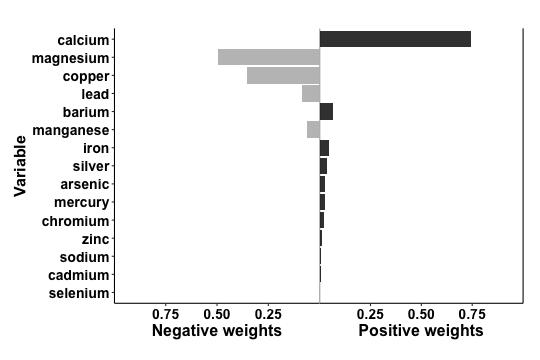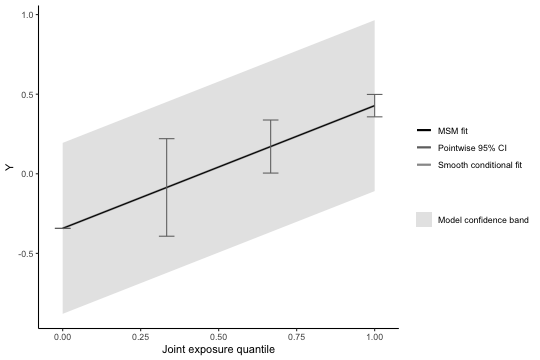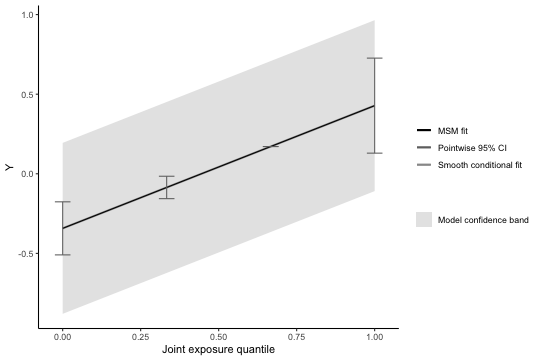From the first plot we see weights from `qgcomp.noboot` function, which include both positive and negative effect directions. When the weights are all on a single side of the null, these plots are easy to in interpret since the weight corresponds to the proportion of the overall effect from each exposure. WQS uses a constraint in the model to force all of the weights to be in the same direction - unfortunately such constraints lead to biased effect estimates. The `qgcomp` package takes a different approach and allows that “weights” might go in either direction, indicating that some exposures may beneficial, and some harmful, or there may be sampling variation due to using small or moderate sample sizes (or, more often, systematic bias such as unmeasured confounding). The “weights” in `qgcomp` correspond to the proportion of the overall effect when all of the exposures have effects in the same direction, but otherwise they correspond to the proportion of the effect in a particular direction, which may be small (or large) compared to the overall “mixture” effect. NOTE: the left and right sides of the plot should not be compared with each other because the length of the bars corresponds to the effect size only relative to other effects in the same direction. The darkness of the bars corresponds to the overall effect size - in this case the bars on the right (positive) side of the plot are darker because the overall “mixture” effect is positive. Thus, the shading allows one to make informal comparisons across the left and right sides: a large, darkly shaded bar indicates a larger independent effect than a large, lightly shaded bar.

Using `qgcomp.boot` also allows us to assess linearity of the total exposure effect (the second plot). Similar output is available for WQS (`gWQS` package), though WQS results will generally be less interpretable when exposure effects are non-linear (see below how to do this with `qgcomp.boot`).

The plot for the `qcboot.fit3` object (using g-computation with bootstrap variance) gives predictions at the joint intervention levels of exposure. It also displays a smoothed (graphical) fit. Generally, we cannot overlay the data over this plot since the regression line corresponds to a change in potentially many exposures at once. Hence, it is useful to explore non-linearity by fitting models that allow for non-linear effects.

### Example 4: non-linearity (and non-homogeneity)

Let's close with one more feature of `qgcomp` (and `qgcomp.boot`): handling non-linearity. Here is an example where we use a feature of the R language for fitting models with interaction terms. We use `y~. + .^2` as the model formula, which fits a model that allows for quadratic term for every predictor in the model.

Similar approaches could be used to include interaction terms between exposures, as well as between exposures and covariates.

``````qcboot.fit4 <- qgcomp(y~. + .^2,
expnms=Xnm,
metals[,c(Xnm, 'y')], family=gaussian(), q=4, B=10, seed=125)
plot(qcboot.fit4)
``````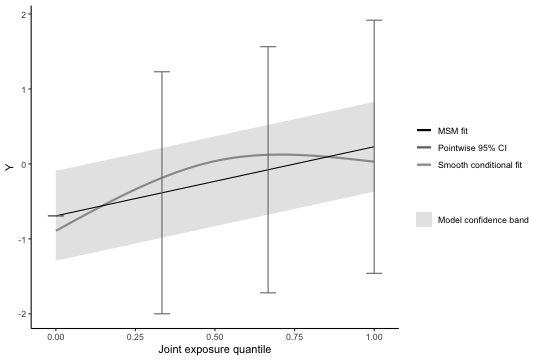Note that allowing for a non-linear effect of all exposures induces an apparent non-linear trend in the overall exposure effect. The smoothed regression line is still well within the confidence bands of the marginal linear model (by default, the overall effect of joint exposure is assumed linear, though this assumption can be relaxed via the 'degree' parameter in qgcomp.boot, as follows:

``````qcboot.fit5 <- qgcomp(y~. + .^2,
expnms=Xnm,
metals[,c(Xnm, 'y')], family=gaussian(), q=4, degree=2,
B=10, rr=FALSE, seed=125)
plot(qcboot.fit5)
``````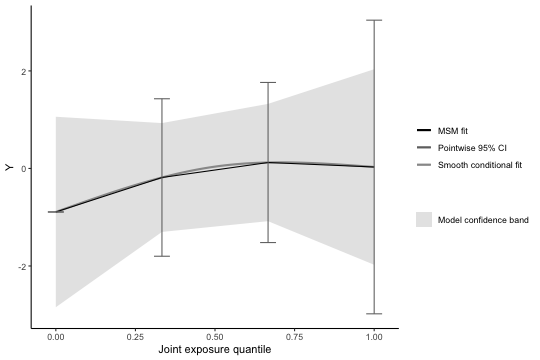Ideally, the smooth fit will look very similar to the model prediction regression line.

### Example 5: Comparing model fits and further exploring non-linearity

Exploring a non-linear fit in settings with multiple exposures is challenging. One way to explore non-linearity, as demonstrated above, is to to include all 2-way interaction terms (including quadratic terms, or “self-interactions”). Sometimes this approach is not desired, either because the number of terms in the model can become very large, or because some sort of model selection procedure is required, which risks inducing over-fit (biased estimates and standard errors that are too small). Short of having a set of a priori non-linear terms to include, we find it best to take a default approach (e.g. taking all second order terms) that doesn't rely on statistical significance, or to simply be honest that the search for a non-linear model is exploratory and shouldn't be relied upon for robust inference. Methods such as kernel machine regression may be good alternatives, or supplementary approaches to exploring non-linearity.

NOTE: qgcomp necessarily fits a regression model with exposures that have a small number of possible values, based on the quantile chosen. By package default, this is `q=4`, but it is difficult to fully examine non-linear fits using only four points, so we recommend exploring larger values of `q`, which will change effect estimates (i.e. the model coefficient implies a smaller change in exposures, so the expected change in the outcome will also decrease).

Here, we examine a couple one strategy for default and exploratory approaches to mixtures that can be implemented in qgcomp using a smaller subset of exposures (iron, lead, cadmium), which we choose via the correlation matrix. High correlations between exposures may result from a common source, so small subsets of the mixture may be useful for examining hypotheses that relate to interventions on a common environmental source or set of behaviors. Note that we can still adjust for the measured exposures, even though only 3 our exposures of interest are considered as the mixture of interest. Note that we will require a new R package to help in exploring non-linearity: `splines`. Note that `qgcomp.boot` must be used in order to produce the graphics below, as `qgcomp.noboot` does not calculate the necessary quantities.

#### Graphical approach to explore non-linearity in a correlated subset of exposures using splines

``````library(splines)
# find all correlations > 0.6 (this is an arbitrary choice)
cormat = cor(metals[,Xnm])
idx = which(cormat>0.6 & cormat <1.0, arr.ind = TRUE)
newXnm = unique(rownames(idx)) # iron, lead, and cadmium

qc.fit6lin <- qgcomp.boot(y ~ iron + lead + cadmium +
mage35 + arsenic + magnesium + manganese + mercury +
selenium + silver + sodium + zinc,
expnms=newXnm,
metals, family=gaussian(), q=8, B=10)

qc.fit6nonlin <- qgcomp.boot(y ~ bs(iron) + bs(cadmium) + bs(lead) +
mage35 + arsenic + magnesium + manganese + mercury +
selenium + silver + sodium + zinc,
expnms=newXnm,
metals, family=gaussian(), q=8, B=10, degree=2)

qc.fit6nonhom <- qgcomp.boot(y ~ bs(iron)*bs(lead) + bs(iron)*bs(cadmium) + bs(lead)*bs(cadmium) +
mage35 + arsenic + magnesium + manganese + mercury +
selenium + silver + sodium + zinc,
expnms=newXnm,
metals, family=gaussian(), q=8, B=10, degree=3)
# it helps to place the plots on a common y-axis, which is easy due
#  to dependence of the qgcomp plotting functions on ggplot
pl.fit6lin <- plot(qc.fit6lin, suppressprint = TRUE, pointwiseref = 4)
pl.fit6nonlin <- plot(qc.fit6nonlin, suppressprint = TRUE, pointwiseref = 4)
pl.fit6nonhom <- plot(qc.fit6nonhom, suppressprint = TRUE, pointwiseref = 4)

pl.fit6lin + coord_cartesian(ylim=c(-0.75, .75)) +
ggtitle("Linear fit: mixture of iron, lead, and cadmium")
pl.fit6nonlin + coord_cartesian(ylim=c(-0.75, .75)) +
ggtitle("Non-linear fit: mixture of iron, lead, and cadmium")
pl.fit6nonhom + coord_cartesian(ylim=c(-0.75, .75)) +
ggtitle("Non-linear, non-homogeneous fit: mixture of iron, lead, and cadmium")
``````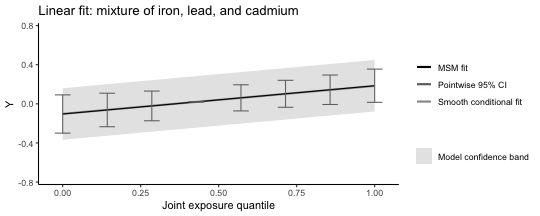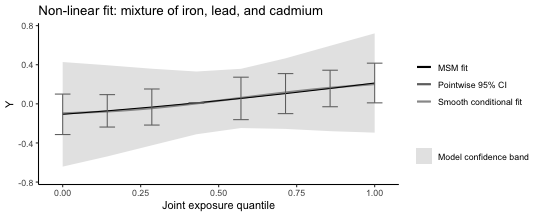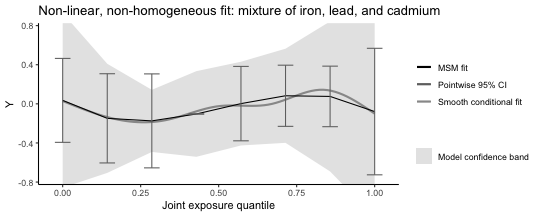#### Caution about graphical approaches

The underlying condtional model fit can be made extremely flexible, and the graphical representation of this (via the smooth conditional fit) can look extremely flexible. Simply matching the overall (MSM) fit to this line is not a viable strategy for identifying parsimonious models because that would ignore potential for overfit. Thus, caution should be used when judging the accuracy of a fit when comparing the “smooth conditional fit” to the “MSM fit.” Here, there is little statistical evidence for even a linear trend, which makes the smoothed conditional fit appear to be overift. The smooth conditional fit can be turned off, as below.

``````qc.overfit <- qgcomp.boot(y ~ bs(iron) + bs(cadmium) + bs(lead) +
mage35 + bs(arsenic) + bs(magnesium) + bs(manganese) + bs(mercury) +
bs(selenium) + bs(silver) + bs(sodium) + bs(zinc),
expnms=Xnm,
metals, family=gaussian(), q=8, B=10, degree=1)
qc.overfit
``````
``````## Mixture slope parameters (bootstrap CI):
##
##              Estimate Std. Error  Lower CI Upper CI t value
## (Intercept) -0.064420   0.123768 -0.307001 0.178162  0.6030
## psi1         0.029869   0.031972 -0.032795 0.092534  0.3507
``````
``````plot(qc.overfit, pointwiseref = 5)
plot(qc.overfit, flexfit = FALSE, pointwiseref = 5)
``````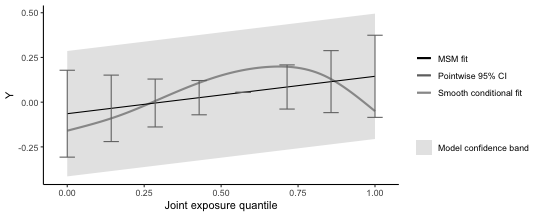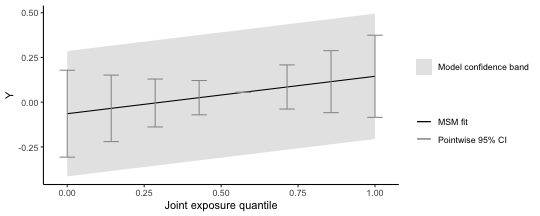### Example 6: Miscellaneous other ways to allow non-linearity. Note that these are included as examples of how to include non-linearities, and are not intended as a demonstration of appropriate model selection.

``````# using indicator terms for each quantile
qc.fit7a <- qgcomp.boot(y ~ factor(iron) + lead + cadmium +
mage35 + arsenic + magnesium + manganese + mercury +
selenium + silver + sodium + zinc,
expnms=newXnm,
metals, family=gaussian(), q=8, B=10, deg=2)
# underlying fit
summary(qc.fit7a\$fit)\$coefficients
``````
``````##                   Estimate Std. Error    t value     Pr(>|t|)
## (Intercept)   -0.052981109 0.08062430 -0.6571357 5.114428e-01
## factor(iron)1  0.046571725 0.09466914  0.4919420 6.230096e-01
## factor(iron)2 -0.056984300 0.09581659 -0.5947227 5.523395e-01
## factor(iron)3  0.143813131 0.09558055  1.5046276 1.331489e-01
## factor(iron)4  0.053319057 0.09642069  0.5529835 5.805601e-01
## factor(iron)5  0.303967859 0.09743261  3.1197753 1.930856e-03
## factor(iron)6  0.246259568 0.09734385  2.5297907 1.176673e-02
## factor(iron)7  0.447045591 0.09786201  4.5681217 6.425565e-06
## lead          -0.009210341 0.01046668 -0.8799679 3.793648e-01
## cadmium       -0.010503041 0.01086440 -0.9667387 3.342143e-01
## mage35         0.081114695 0.07274583  1.1150426 2.654507e-01
## arsenic        0.021755516 0.02605850  0.8348720 4.042502e-01
## magnesium     -0.010758356 0.02469893 -0.4355798 6.633587e-01
## manganese      0.004418266 0.02551449  0.1731670 8.626011e-01
## mercury        0.003913896 0.02448078  0.1598763 8.730531e-01
## selenium      -0.058085344 0.05714805 -1.0164012 3.100059e-01
## silver         0.020971562 0.02407397  0.8711302 3.841658e-01
## sodium        -0.062086322 0.02404447 -2.5821454 1.014626e-02
## zinc           0.017078438 0.02392381  0.7138679 4.756935e-01
``````
``````plot(qc.fit7a)

# interactions between indicator terms
qc.fit7b <- qgcomp.boot(y ~ factor(iron)*factor(lead) + cadmium +
mage35 + arsenic + magnesium + manganese + mercury +
selenium + silver + sodium + zinc,
expnms=newXnm,
metals, family=gaussian(), q=8, B=10, deg=3)
# underlying fit
#summary(qc.fit7b\$fit)\$coefficients
plot(qc.fit7b)

# breaks at specific quantiles (these breaks act on the quantized basis)
qc.fit7c <- qgcomp.boot(y ~ I(iron>3)*I(lead>3) + cadmium +
mage35 + arsenic + magnesium + manganese + mercury +
selenium + silver + sodium + zinc,
expnms=newXnm,
metals, family=gaussian(), q=8, B=10, deg=2)
# underlying fit
summary(qc.fit7b\$fit)\$coefficients
``````
``````##                                 Estimate Std. Error     t value   Pr(>|t|)
## (Intercept)                  0.008649255 0.18245123  0.04740585 0.96221480
## factor(iron)1                0.038268741 0.26368324  0.14513149 0.88468441
## factor(iron)2               -0.345584575 0.27565973 -1.25366360 0.21073911
## factor(iron)3                0.535815627 0.31059571  1.72512241 0.08532276
## factor(iron)4               -0.087345394 0.29520376 -0.29588172 0.76748289
## factor(iron)5                0.323493619 0.23713625  1.36416773 0.17332645
## factor(iron)6               -0.017792547 0.24070452 -0.07391862 0.94111424
## factor(iron)7                0.408858950 0.27605519  1.48107685 0.13941915
## factor(lead)1               -0.162742949 0.25365039 -0.64160339 0.52151959
## factor(lead)2               -0.102463858 0.24030538 -0.42639020 0.67006625
## factor(lead)3                0.101759855 0.26315294  0.38669474 0.69919981
## factor(lead)4               -0.267059281 0.27367740 -0.97581782 0.32977882
## factor(lead)5               -0.151458350 0.26026039 -0.58194930 0.56094774
## factor(lead)6                0.012187149 0.26552532  0.04589826 0.96341558
## factor(lead)7               -0.303835377 0.31203911 -0.97370928 0.33082360
## cadmium                     -0.008264363 0.01243054 -0.66484338 0.50655607
## mage35                       0.050512906 0.07819749  0.64596584 0.51869343
## arsenic                      0.010412309 0.02761714  0.37702342 0.70636777
## magnesium                   -0.024437647 0.02641175 -0.92525669 0.35542271
## manganese                    0.011518263 0.02739649  0.42042842 0.67441141
## mercury                      0.013546992 0.02682344  0.50504311 0.61382288
## selenium                    -0.040476677 0.06255534 -0.64705392 0.51798977
## silver                       0.005222418 0.02618757  0.19942355 0.84203867
## sodium                      -0.057193337 0.02648343 -2.15958943 0.03143279
## zinc                         0.027475973 0.02546297  1.07905608 0.28125078
## factor(iron)1:factor(lead)1 -0.021692958 0.39301568 -0.05519616 0.95601135
## factor(iron)2:factor(lead)1  0.274143816 0.38649262  0.70931190 0.47856821
## factor(iron)3:factor(lead)1 -0.509139502 0.42361419 -1.20189436 0.23015689
## factor(iron)4:factor(lead)1  0.152395343 0.39685706  0.38400562 0.70119019
## factor(iron)5:factor(lead)1 -0.302326717 0.35223539 -0.85830874 0.39126592
## factor(iron)6:factor(lead)1  0.630416417 0.33903064  1.85946739 0.06373766
## factor(iron)7:factor(lead)1  0.053602296 0.37274385  0.14380464 0.88573137
## factor(iron)1:factor(lead)2  0.024337827 0.34857026  0.06982187 0.94437236
## factor(iron)2:factor(lead)2  0.538907789 0.36386593  1.48106143 0.13942326
## factor(iron)3:factor(lead)2 -0.258506137 0.41620361 -0.62110498 0.53490490
## factor(iron)4:factor(lead)2  0.243703555 0.38673044  0.63016388 0.52896812
## factor(iron)5:factor(lead)2 -0.424129769 0.45529160 -0.93155633 0.35216045
## factor(iron)6:factor(lead)2  0.296886216 0.38166057  0.77788024 0.43712622
## factor(iron)7:factor(lead)2  0.259315114 0.35440575  0.73168991 0.46481122
## factor(iron)1:factor(lead)3 -0.355619483 0.36456769 -0.97545529 0.32995830
## factor(iron)2:factor(lead)3  0.345643942 0.39749814  0.86954859 0.38509895
## factor(iron)3:factor(lead)3 -0.664859527 0.41325017 -1.60885482 0.10848292
## factor(iron)4:factor(lead)3 -0.265634335 0.40425063 -0.65710308 0.51151452
## factor(iron)5:factor(lead)3 -0.305504688 0.36946853 -0.82687608 0.40882896
## factor(iron)6:factor(lead)3  0.247675270 0.38136238  0.64944862 0.51644288
## factor(iron)7:factor(lead)3 -0.004014205 0.36765547 -0.01091839 0.99129432
## factor(iron)1:factor(lead)4  0.075037453 0.37862341  0.19818493 0.84300692
## factor(iron)2:factor(lead)4  0.029417826 0.44850364  0.06559105 0.94773809
## factor(iron)3:factor(lead)4 -0.397223331 0.39869070 -0.99631953 0.31973227
## factor(iron)4:factor(lead)4  0.565411002 0.38829274  1.45614621 0.14618227
## factor(iron)5:factor(lead)4  0.604065345 0.37727475  1.60112849 0.11018382
## factor(iron)6:factor(lead)4  0.530855883 0.37819173  1.40366865 0.16123852
## factor(iron)7:factor(lead)4  0.156270989 0.44814735  0.34870448 0.72750532
## factor(iron)1:factor(lead)5  0.166787844 0.37753784  0.44177782 0.65890261
## factor(iron)2:factor(lead)5  0.270272970 0.37220075  0.72614838 0.46819719
## factor(iron)3:factor(lead)5 -0.438011462 0.40273235 -1.08759940 0.27746499
## factor(iron)4:factor(lead)5 -0.033176270 0.40442824 -0.08203253 0.93466427
## factor(iron)5:factor(lead)5 -0.084517743 0.34793903 -0.24290963 0.80820716
## factor(iron)6:factor(lead)5  0.463147899 0.39543283  1.17124292 0.24223892
## factor(iron)7:factor(lead)5 -0.181509765 0.39461755 -0.45996374 0.64580670
## factor(iron)1:factor(lead)6  0.063358911 0.38612410  0.16408950 0.86974839
## factor(iron)2:factor(lead)6  0.307049953 0.37177377  0.82590536 0.40937874
## factor(iron)3:factor(lead)6 -0.244578103 0.41416001 -0.59054012 0.55518149
## factor(iron)4:factor(lead)6 -0.048117136 0.38927385 -0.12360742 0.90169182
## factor(iron)5:factor(lead)6 -0.123437997 0.37008045 -0.33354368 0.73890887
## factor(iron)6:factor(lead)6 -0.215630812 0.38081363 -0.56623711 0.57156862
## factor(iron)7:factor(lead)6 -0.089645370 0.40467009 -0.22152705 0.82480170
## factor(iron)1:factor(lead)7  0.517772174 0.50884115  1.01755170 0.30954188
## factor(iron)2:factor(lead)7  0.337874279 0.41472279  0.81469909 0.41575755
## factor(iron)3:factor(lead)7 -0.192842373 0.43833835 -0.43993954 0.66023233
## factor(iron)4:factor(lead)7  0.424740851 0.44386234  0.95692022 0.33921913
## factor(iron)5:factor(lead)7  0.320125740 0.38694521  0.82731542 0.40858027
## factor(iron)6:factor(lead)7  0.315511296 0.38782433  0.81354178 0.41641965
## factor(iron)7:factor(lead)7 -0.014406334 0.41525519 -0.03469272 0.97234308
``````
``````plot(qc.fit7c)
``````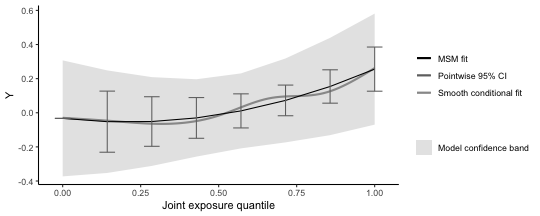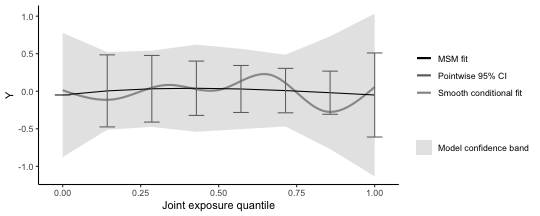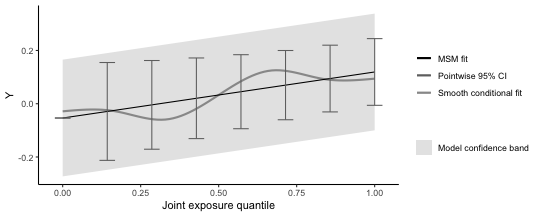Note one restriction on exploring non-linearity: while we can use flexible function such as splines for individual exposures, the overall fit is limited via the `degree` parameter to polynomial functions (here a quadratic polynomial fits the non-linear model well, and a cubic polynomial fits the non-linear/non-homogenous model well - though this is an informal argument and does not account for the wide confidence intervals). We note here that only 10 bootstrap iterations are used to calculate confidence intervals (to increase computational speed for the xample), which is far too low.

#### Statistical approach explore non-linearity in a correlated subset of exposures using splines

The graphical approaches don't give a clear picture of which model might be preferred, but we can compare the model fits using AIC, AICC, or BIC (all various forms of information criterion that weigh model fit with over-parameterization). Both of these criterion suggest the linear model fits best (lowest AIC and BIC), which suggests that the apparently non-linear fits observed in the graphical approaches don't improve prediction of the health outcome, relative to the linear fit, due to the increase in variance associated with including more parameters.

``````AIC(qc.fit6lin\$fit)
``````
``````##  676.0431
``````
``````AIC(qc.fit6nonlin\$fit)
``````
``````##  682.7442
``````
``````AIC(qc.fit6nonhom\$fit)
``````
``````##  705.6187
``````
``````BIC(qc.fit6lin\$fit)
``````
``````##  733.6346
``````
``````BIC(qc.fit6nonlin\$fit)
``````
``````##  765.0178
``````
``````BIC(qc.fit6nonhom\$fit)
``````
``````##  898.9617
``````

### Example 7: time-to-event analysis and parallel processing

• The `qgcomp` package utilizes Cox proportional hazards models as the underlying model for time-to-event analysis. The interpretation of a `qgcomp.noboot` fit parameter is a conditional (on confounders) hazard ratio for increasing all exposures at once. The `qc.survfit1` object demonstrates a time-to- event analysis with `qgcompcox.noboot`. The default plot is similar to that of `qgcompcox.noboot`, in that it yields weights and an overall mixture effect
• Marginal hazards ratios (and bootstrapped quantile g-computation in general) uses a slightly different approach to effect estimation that makes it more computationally demanding than other `qcomp` functions. To estimate a marginal hazards ratio, the underlying model is fit, and then new outcomes are simulated under the underlying model with a baseline hazard estimator (Efron's) - this simulation requires a large sample (controlled by MCsize) for accuracy. This approach is similar to other g-computation approaches to survival analysis, but this approach uses the exact survival times, rather than discretized survival times as are common in most g-computation analysis. Plotting a `qgcompcox.boot` object yields a set of survival curves (e.g.`qc.survfit2`) which comprise estimated survival curves (assuming censoring and late entry at random, conditional on covariates in the model) that characterize conditional survival functions (i.e. censoring competing risks) at various levels of joint-exposure (including the overall average - which may be slightly different from the observed survival curve, but should more or less agree).
• All bootstrapped functions in `qgcomp` allow parellelization via the parallel=TRUE parameter (demonstrated with the non-liner fit in`qc.survfit3`). Only 5 bootstrap iterations are used here, which is not nearly enough for inference, and will actually be slower for parallel processing due to some overhead when setting up the parallel processes.
• While`qgcompcox.boot` fits a smooth hazard ratio function, the hazard ratios contrasting specific quantiles with a referent quantile can be obtained, as demonstrated with `qc.survfit4`. As in `qgcomp.boot` plots, the conditional model fit and the MSM fit are overlaid as a way to judge how well the MSM fits the conditional fit (and whether, for example non-linear terms should be added or removed from the overall fit via the degree parameter - we note here that we know of no statistical test for quantifying the difference between these lines, so this is up to user discretion and the plots are provided as visuals to aid in exploratory data analysis).
``````# non-bootstrapped version estimates a marginal structural model for the
# confounder-conditional effect
survival::coxph(survival::Surv(disease_time, disease_state) ~ iron + lead + cadmium +
arsenic + magnesium + manganese + mercury +
selenium + silver + sodium + zinc +
mage35,
data=metals)
``````
``````## Call:
## survival::coxph(formula = survival::Surv(disease_time, disease_state) ~
##     iron + lead + cadmium + arsenic + magnesium + manganese +
##         mercury + selenium + silver + sodium + zinc + mage35,
##     data = metals)
##
##                coef exp(coef)  se(coef)      z      p
## iron      -0.056447  0.945117  0.156178 -0.361 0.7178
## lead       0.440735  1.553849  0.203264  2.168 0.0301
## cadmium    0.023325  1.023599  0.105502  0.221 0.8250
## arsenic   -0.003812  0.996195  0.088897 -0.043 0.9658
## magnesium  0.099399  1.104507  0.064730  1.536 0.1246
## manganese -0.014065  0.986033  0.064197 -0.219 0.8266
## mercury   -0.060830  0.940983  0.072918 -0.834 0.4042
## selenium  -0.231626  0.793243  0.173655 -1.334 0.1823
## silver     0.043169  1.044114  0.070291  0.614 0.5391
## sodium     0.057928  1.059638  0.063883  0.907 0.3645
## zinc       0.057169  1.058835  0.047875  1.194 0.2324
## mage35    -0.458696  0.632107  0.238370 -1.924 0.0543
##
## Likelihood ratio test=23.52  on 12 df, p=0.02364
## n= 452, number of events= 205
``````
``````qc.survfit1 <- qgcomp.cox.noboot(survival::Surv(disease_time, disease_state) ~ .,expnms=Xnm,
data=metals[,c(Xnm, 'disease_time', 'disease_state')], q=4)
qc.survfit1
``````
``````## Mixture log(hazard ratio) (Delta method CI):
##
##      Estimate Std. Error Lower CI Upper CI Z value Pr(>|z|)
## psi1 -0.23356    0.24535 -0.71444  0.24732 -0.9519   0.3411
``````
``````plot(qc.survfit1)

# bootstrapped version estimates a marginal structural model for the population average effect
#library(survival)
qc.survfit2 <- qgcomp.cox.boot(Surv(disease_time, disease_state) ~ .,expnms=Xnm,
data=metals[,c(Xnm, 'disease_time', 'disease_state')], q=4,
B=5, MCsize=1000, parallel=TRUE)
``````
``````## Expected time to finish: 0.63 minutes
``````
``````qc.survfit2
``````
``````## Mixture log(hazard ratio) (bootstrap CI):
##
##      Estimate Std. Error Lower CI Upper CI Z value Pr(>|z|)
## psi1 -0.23829    0.18939 -0.60948   0.1329 -1.2582   0.2083
``````
``````p2 = plot(qc.survfit2, suppressprint = TRUE)
p2 + labs(title="Linear log(hazard ratio), overall and exposure specific")

qc.survfit3 <- qgcomp.cox.boot(Surv(disease_time, disease_state) ~ . + .^2,expnms=Xnm,
data=metals[,c(Xnm, 'disease_time', 'disease_state')], q=4,
B=5, MCsize=1000, parallel=TRUE)
``````
``````## Expected time to finish: 0.64 minutes
``````
``````qc.survfit3
``````
``````## Mixture log(hazard ratio) (bootstrap CI):
##
##      Estimate Std. Error Lower CI Upper CI Z value Pr(>|z|)
## psi1 -0.21323    0.33608 -0.87193  0.44548 -0.6345   0.5258
``````
``````p3 = plot(qc.survfit3, suppressprint = TRUE)
p3 + labs(title="Non-linear log(hazard ratio) overall, linear exposure specific ln-HR")

qc.survfit4 <- qgcomp.cox.boot(Surv(disease_time, disease_state) ~ . + .^2,expnms=Xnm,
data=metals[,c(Xnm, 'disease_time', 'disease_state')], q=4,
B=5, MCsize=1000, parallel=TRUE, degree=2)
``````
``````## Expected time to finish: 0.62 minutes
``````
``````## Warning in fitter(X, Y, istrat, offset, init, control, weights = weights, :
## Loglik converged before variable 1,2 ; coefficient may be infinite.
``````
``````qc.survfit4
``````
``````## Mixture log(hazard ratio) (bootstrap CI):
##
##      Estimate Std. Error Lower CI Upper CI Z value Pr(>|z|)
## psi1 -2.72549   15.72000 -33.5361   28.085 -0.1734   0.8624
## psi2  0.83276    5.19763  -9.3544   11.020  0.1602   0.8727
``````
``````# examining the overall hazard ratio as a function of overall exposure
hrs_q = exp(matrix(c(0,0,1,1,2,4,3,9), ncol=2, byrow=TRUE)%*%qc.survfit4\$msmfit\$coefficients)
colnames(hrs_q) = "Hazard ratio"
print("Hazard ratios by quartiles (min-25%,25-50%, 50-75%, 75%-max)")
``````
``````##  "Hazard ratios by quartiles (min-25%,25-50%, 50-75%, 75%-max)"
``````
``````hrs_q
``````
``````##      Hazard ratio
## [1,]    1.0000000
## [2,]    0.1506586
## [3,]    0.1200356
## [4,]    0.5057654
``````
``````p4 = plot(qc.survfit4, suppressprint = TRUE)
p4 + labs(title="Non-linear log(hazard ratio), overall and exposure specific")
``````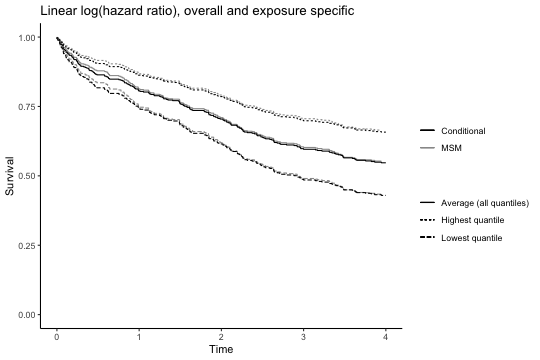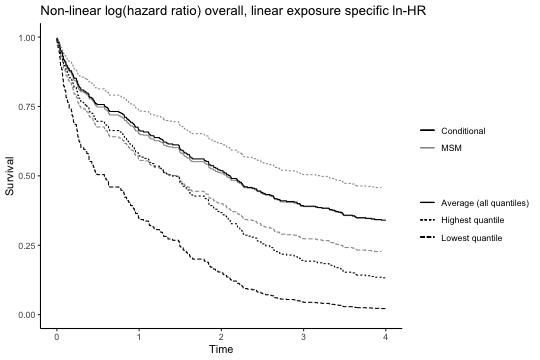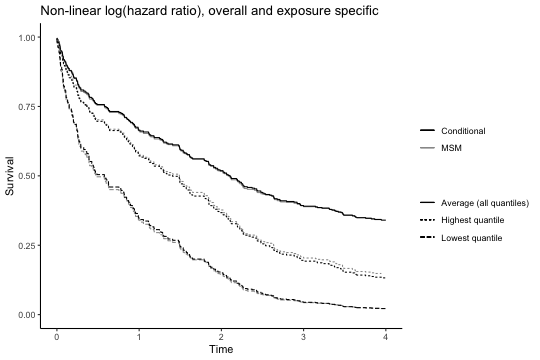## References

Alexander P. Keil, Jessie P. Buckley, Katie M. O’Brien, Kelly K. Ferguson, Shanshan Zhao, Alexandra J. White. A quantile-based g-computation approach to addressing the effects of exposure mixtures. https://arxiv.org/abs/1902.04200

## Acknowledgements

The development of this package was supported by NIH Grant RO1ES02953101. Invaluable code testing was performed by Nicole Niehoff, Michiel van den Dries, Emily Werder, Jessie Buckley, and Katie O'Brien.